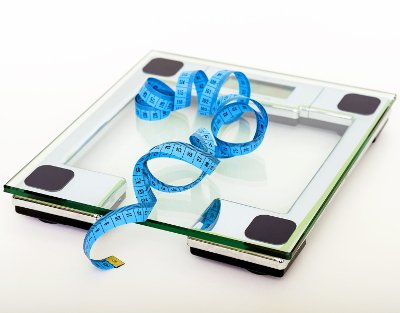# Ideal Body Weight (IBW) / Healthy Weight Calculator

Share:

Ideal Body Weight or IBW is the expected or desired weight of the human body corresponding to the height. IBW is otherwise called as Healthy Weight.

Select your gender, enter your height and select the formula to calculate. Then click the "Calculate IBW" button.

*
*
ft
Feet
in
Inches
cm
Centimeters

Similar Fitness Calculators

## Ideal Body Weight (IBW) Calculator / Healthy Weight CalculatorThis IBW calculator takes your height and gender and calculates your ideal body weight in kilograms and pounds. You have the option to select the IBW formula you want the Ideal Body Weight to be calculated.

### Using the Ideal Body Weight calculator

2. Enter your Height either in centimeters or in feet.
3. Select the ideal body weight formula. By default, J. D. Robinson formula will be selected. If needed you can choose other formulas as well.
4. Then, click the Calculate IBW button. The ideal body weight will be calculated and the result will be displayed in the box below the button. The ideal body weight will be both in kilograms and pounds. The ideal body weight calculated using the BMI formula will be a range of minimum and maximum ideal weight.

## Ideal Body Weight (IBW)

There are several formulas to calculate ideal human body weight. The popular once are given below. These formulas are based on the height and gender.

### Ideal Body Weight Formulas For Men and Women

#### 1. J. D. Robinson Formula:

IBW (For Men)= 52 + (1.9 x (Ht - 60))

IBW (For Women)= 49 + (1.7 x (Ht - 60))

Where:

• IBW = Ideal Body Weight in Kilograms (Kg).
• Ht = height in Inches (in.).

#### 2. D. R. Miller Formula:

IBW (For Men)= 56.2 + (1.41 x (Ht - 60))

IBW (For Women)= 53.1 + (1.36 x (Ht - 60))

Where:

• IBW = Ideal Body Weight in Kilograms (Kg).
• Ht = height in Inches (in.).

#### 3. G. J. Hamwi Formula:

IBW (For Men)= 48 + (2.7 x (Ht - 60))

IBW (For Women)= 45.5 + (2.2 x (Ht - 60))

Where:

• IBW = Ideal Body Weight in Kilograms (Kg).
• Ht = height in Inches (in.).

#### 4. B. J. Devine Formula:

IBW (For Men)= 50 + (2.3 x (Ht - 60))

IBW (For Women)= 45.5 + (2.3 x (Ht - 60))

Where:

• IBW = Ideal Body Weight in Kilograms (Kg).
• Ht = height in Inches (in.).

#### 5. Body Mass Index Formula:

IBW (Minimum)= (18.5 ÷ 703) x Ht2

IBW (Maximum)= (24.9 ÷ 703) x Ht2

Where:

• IBW = Ideal Body Weight in Kilograms (Kg).
• Ht = height in Inches (in.).